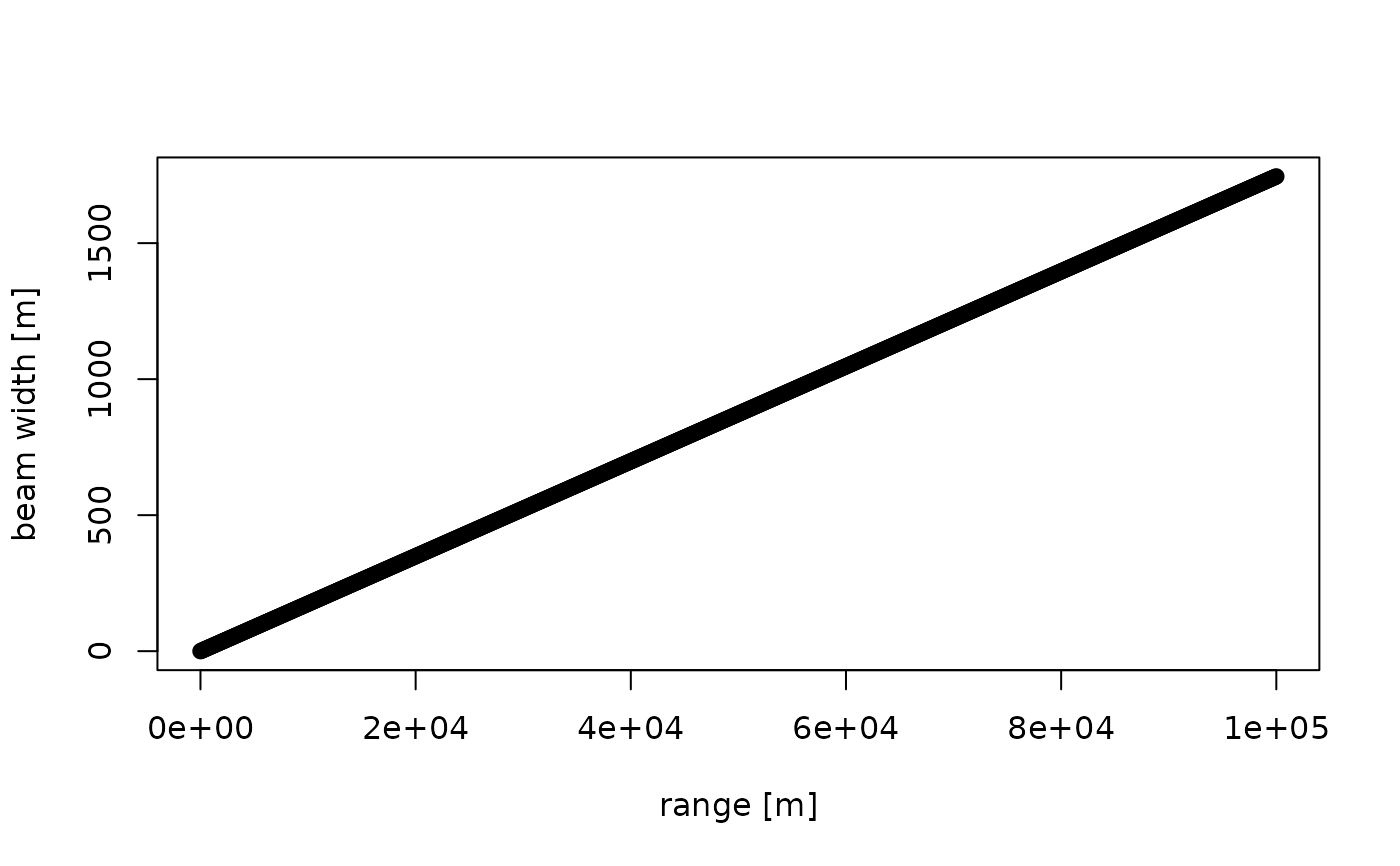Calculates the width of a radar beam as a function of range and beam angle.

beam_width(range, beam_angle = 1)

## Arguments

range

numeric. Range (distance from the radar antenna) in m.

beam_angle

numeric. Beam opening angle in degrees, typically the angle between the half-power (-3 dB) points of the main lobe

## Value

numeric. Beam width in m.

## Examples

#' # beam width in meters at 10 km range:
beam_width(10000)
#>  174.5241

# define ranges from 0 to 1000000 meter (100 km), in steps of 100 m:
range <- seq(0, 100000, 100)

# plot the beam width as a function of range:
plot(range, beam_width(range), ylab = "beam width [m]", xlab = "range [m]")